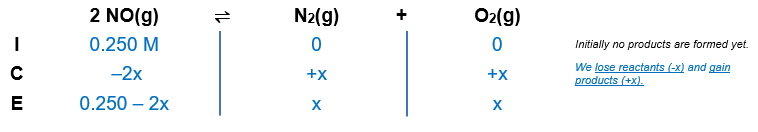# Problem: At 2000°C the equilibrium constant for the reaction2NO(g) ⇋ N2(g)+O2(g)is Kc=2.4×103. The initial concentration of NO is 0.250 M.What is the equilibrium concentration of NO?What is the equilibrium concentration of N2?What is the equilibrium concentration of O2?

###### FREE Expert Solution

Given:

[NO] = 0.250 M

2 NO(g) ⇌ N2(g)+O2(g)

is Kc = 2.4×103

Balanced chemical equation: 2 NO(g) ⇌ N2(g)+O2(g)

Step 1: Create an ICE chart.Step 2: Solve for x.

91% (474 ratings)###### Problem Details

At 2000°C the equilibrium constant for the reaction

2NO(g) ⇋ N2(g)+O2(g)

is Kc=2.4×103. The initial concentration of NO is 0.250 M.

What is the equilibrium concentration of NO?

What is the equilibrium concentration of N2?

What is the equilibrium concentration of O2?